• 大物实验-密立根油滴实验，实验数据处理，这个实验数据处理起来太麻烦就写了个程序计算，不用自己一遍遍算，用Visual Basic(VB)写的，运行那个exe文件就能用，很方便
• 基于MATLAB的密立根油滴实验数据处理软件.pdf
• 这是大学物理实验的密立根油滴实验数据处理的软件！很简洁实用的一个软件，不用复杂的公式，只要把测得的数据写进去，然后点击计算，一切家ok！可啊！！
• Matlab在密立根油滴实验数据处理中的应用.pdf
• 大学物理实验中密立根油滴实验数据处理用C++实现的方法！
• 密立根油滴实验数据处理c语言代码，这个代码经过我的反复修改与检验，其处理数据的准确度大幅提高
• 实验数据 大学物理 cdd密立根油滴实验数据处理 含部分数据
• 密立根油滴实验数据处理表格（北理） 请在下降时间和平衡电压栏输入,在电量和电荷数栏查看结果.
• 基于python对密立根油滴实验数据处理改进 近似最大公约数 import math ac=1.34 def accuracy(x, y): return math.fabs(x - y) < ac def gcd(x, y): if accuracy(x, 0): return y if accuracy(y, 0): return ...
基于python对密立根油滴实验数据处理改进
近似最大公约数
import math
ac=1.34
def accuracy(x, y):
return math.fabs(x - y) < ac
def gcd(x, y):
if accuracy(x, 0):
return y
if accuracy(y, 0):
return x
return gcd(y, x%y)

b=[3.2,3.2,6.4,4.8,8.0,9.6]
a=[1.60217663410,2*1.60217663410,3*1.60217663410,4*1.60217663410,5*1.60217663410,6*1.60217663410]
c=[7.88,6.25,3.11,3.18,4.57,6.29]
d=[9.36,3.16,3.41,10.78,4.90,3.11]
x=[7.88,6.25,3.11,3.18,4.57,6.29]
f=[2*1.60217663410,100,3*1.60217663410,4*1.60217663410,5*1.60217663410,6*1.60217663410]
n=6
e=2*1.60217663410
for j in range(0,10):
for i in range(1,n):
e=gcd(e, a[i])
for i in range(1,n):
if(a[i]%e>10):
del a[i]
n=n-1
print(e)

梯度下降拟合“倒过来验证”方法
import numpy as np
import matplotlib.pyplot as plt

N = 6
x = np.array([5,4,2,2,3,4]).reshape(N,1)
y = np.array([7.88,6.25,3.11,3.18,4.57,6.29]).reshape(N,1)
plt.scatter(x, y)
ones = np.ones((N, 1))
x = np.hstack((x, ones))

diff = np.dot(x, theta) - y

def Iteration():
alpha = 0.001
theta = np.array([1.6, 0]).reshape(2, 1)
epsilon = 0.001
theta = theta - alpha * gradient
return theta

Right_theta = Iteration()
print('下降后e值为：')
print('e=',Right_theta)
print('拟合函数为:')
print('y=',Right_theta,'x')

x1 = np.linspace(1, 10, 100)
y1 = Right_theta*x1 + Right_theta
plt.plot(x1, y1)
plt.show()



展开全文• 很实用的数据处理项目，大学物理必备的环节，可以让你在实验室之外随意尝试C语言
• omwin1.7密立根油滴实验处理数据软件。输入提升电压，油滴下降时间和上升时间可以自动进行数据计算出电荷量等
• 针对应用于密立根实验数据处理中的“倒过来验证法”的缺点，文章提出了一种新的数据处理方法，使数据处理结果能够直接、真正地反映实验目的。
• 密立根油滴实验实验数据处理 要求写计算过程，其中数据可由此程序计算，其他可以随便写点过程（2333 #include<stdio.h> #include<stdlib.h> #include<math.h> #define Pi 3.14159265359 //定义...
密立根油滴实验实验数据处理
要求写计算过程，其中数据可由此程序计算，其他可以随便写点过程（2333
#include<stdio.h>
#include<stdlib.h>
#include<math.h>
#define Pi 3.14159265359      //定义圆周率的取值
#define DENSITY 981       //油滴的密度
#define b (6.17e-6)       //粘滞系数修正常数
#define g 9.801           //重力加速度
#define d 5e-3            //极板间距离
#define l (1.5e-3)        //油滴匀速上升或下降的距离
#define p 76              //大气压强
#define e 1.60217733e-19         //元电荷
#define ETA (1.83e-5)     //粘滞系数
int main(){
float q,U,tg,a,q1,x,te,k,vg,ve,s,y;
int i,t,n,n2;
while (2>1)	{
printf("平衡法请输入1，动态法请输入2，计算7组数据的平均值请输入3，结束程序请输入其他数字\n");
scanf("%d",&t);
if (t==1){
printf("请输入平衡电压U和下降时间tg，用空格隔开\n");
scanf("%f %f",&U,&tg);
a = sqrt((9*ETA*l)/(2*DENSITY*g*tg));
k = ((18*Pi*d)/(sqrt(2*DENSITY*g)))*pow((ETA*l)/((1+(b/(p*a)))),1.5);
vg = l/tg;
q=k/U*pow((1/tg),1.5);
q1=q/e;
n=q1;
if (q1-n>0.49)
n++;
q1=q/n;
x=abs((q1-e)*10000/e);
printf("平衡法总电荷值：%e\n电子数：%d\n电子平均电荷值：%e\n下降速度vg: %e\n与元电荷的误差：%3.2f%%\n\n",q,n,q1,vg,x/100);
}
else if (t==2){
printf("请输入提升电压U、下降时间tg和上升时间te，用空格隔开\n");
scanf("%f %f %f",&U,&tg,&te);
a = sqrt((9*ETA*l)/(2*DENSITY*g*tg));
k = ((18*Pi*d)/(sqrt(2*DENSITY*g)))*pow((ETA*l)/((1+(b/(p*a)))),1.5);
vg = l/tg;
ve = l/te;
q=k/U*(1/tg+1/te)*sqrt(1/tg);
q1=q/e;
n=q1;
if(q1-n>0.49)
n++;
q1=q/n;
x=abs((q1-e)*10000/e);
printf("动态法总电荷值：%e\n电子数：%d\n电子平均电荷值：%e\n下降速度vg: %e\n上升速度ve: %e\n待求值a: %e\n待求值K :%e\n与元电荷的误差：%3.2f%%\n\n",q,n,q1,vg,ve,a,k,x/100);
}
else if (t==3){
printf("请输入7个数据\n");
s=0;
for (i=1;i<=7;i++){
scanf("%f",&y);
s+=y;
}
s=s/7;
printf("平均值为： %f\n",s);
}
else
break;
}
}




展开全文• 不知道你们的实验数据处理是不是这样子的呢，我们的要求是测得三滴油滴每个油滴测量五次 由上式计算被测油滴所带电量，并用“倒算法”求出油滴所带电荷个数 ( e0 ＝1．60×10-19C ) 并将n取整数值N，再用这个整数N...
不知道你们的实验数据处理是不是这样子的呢，我们的要求是测得三滴油滴每个油滴测量五次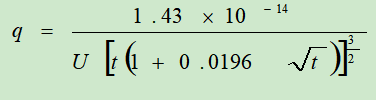由上式计算被测油滴所带电量，并用“倒算法”求出油滴所带电荷个数
(   e0   ＝1．60×10-19C  )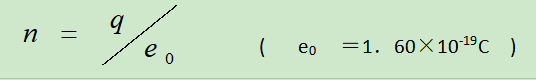并将n取整数值N，再用这个整数N去除所测得的电量值，得到测得的基本电荷值。
求得电子电荷e的平均值，并于  比较，计算相对误差，写出结果表达式。
![在这里插入图片描述](https://img-blog.csdnimg.cn/20200526143655978.png![在这里插入图片描述](https://img-blog.csdnimg.cn/20200526143707531.png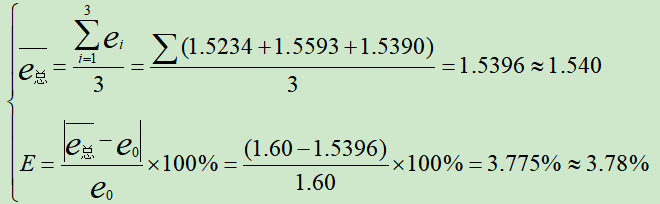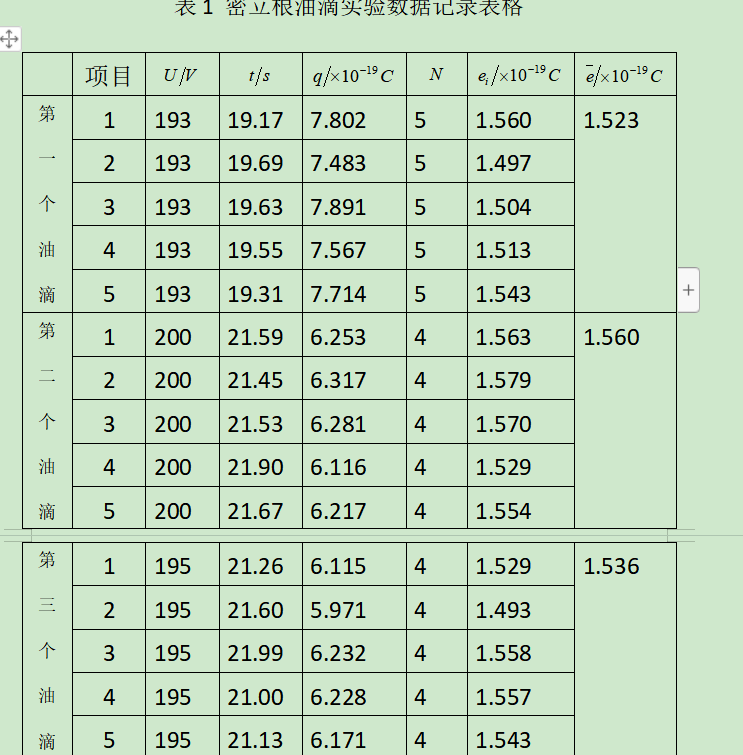附上代码 秒出结果
# include<iostream>
# include<cmath>
using namespace std;

//自定义函数四舍五入
int round(float f)
{
if ((int)f+0.5>f)
return (int)f;
else
return (int)f + 1;
}

int main()
{
double u;
int i;
double t;      //数组可以赋值也可以不赋值//={0.0};
double e;
double n;
int N;
long double q;
double sum1,sum2;
double e_Ever;       // c++定义数组必须给出大长度
double e_Zhong_Ever;
double Avery;
double E;
double e0=1.60e-19;
double long xiangdui;
cout<<"请输入此次测量电压(输入完电压按回车即可):"<<endl;
sum2=0;
for(int j=1;j<5;j++)
{
sum1=0;

cin>>u;
cout<<"请输入此次测量五次时间(以空格形式分开):"<<endl;
for(i=0;i<5;i++)
{
cin>>t[i];
q=(1.43e-14)/(u* pow(   (t[i]*(1+0.0196*pow(t[i],0.5))) , 1.5)  );
n=q/1.60e-19;
N=round(n);
e[i]=q/N;

cout<<"第"<<i+1<<"次测量q为："<<q<<" ";

cout<<"  m为："<<N;
cout<<"  e为："<<e[i]<<endl<<endl;

sum1 += e[i];
e_Ever[i]=sum1/(i+1);        //只能说它代表平均值
Avery=e_Ever[i];
}

cout<<"  e平均值为："<<Avery<<endl;
sum2 += Avery;

e_Zhong_Ever=sum2/3;     cout<<"  e最终平均值为："<<e_Zhong_Ever;
E=(fabs(e_Zhong_Ever-e0))/1.60e-19;	cout<<"  E相对误差："<<E*100<<'%'<<endl;

if(j<3)
{cout<<"请输入第"<<j+1<<"次测量电压:"<<endl;}

}

int a=abs(213-3);cout<<"a="<<a;
return 0;
}


附上结果：（表格里的所有数都就出来啦）
由于我水平有限，e最终平均值和E相对误差看最后一次的就好啦，所以不要有疑惑哦！（其实是因为作用域的问题，但我怎么改也改不好啦，所以就先这样吧！）再说一遍，由于我水平有限，e最终平均值和E相对误差看最后一次的就好啦，所以不要有疑惑哦！**
也就是下面这个样子了，*
注意注意：也就是不看第一次和第二次测得的e最终平均值和E相对误差,只看最后一次的就好啦*
不看画“×”的（忽略就好），只看最后一次画“√”的就好了，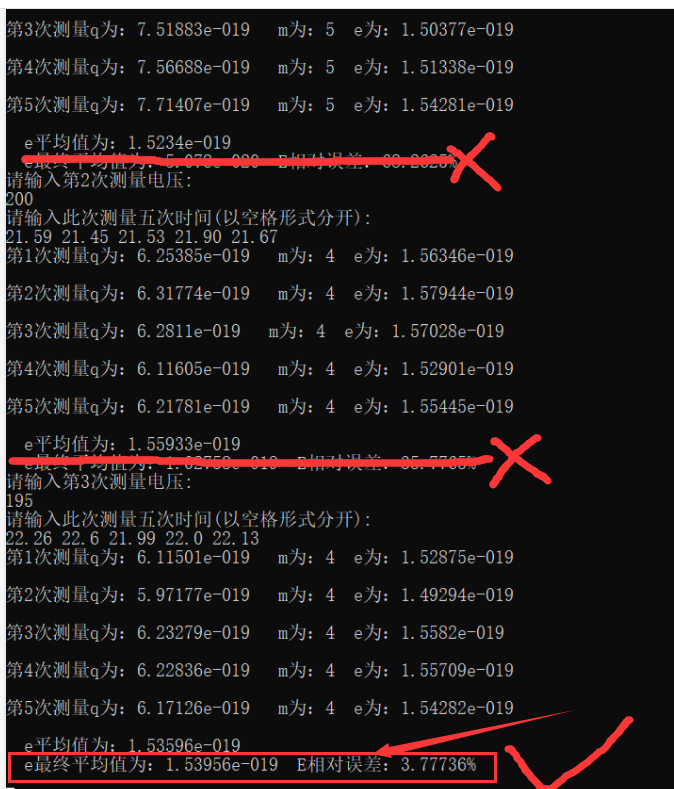其实看到这里也就结束啦！如果你跟我的处理方式一样一样，解决这个实验数据处理够用啦！
———————————————————假设有分割线————————————————
1题外话：不知道你有没有发现我是自定义的四舍五入函数？
原因是，我在网上查找的四舍五入是这样的：
在math.h或cmath头文件中有四舍五入有关的函数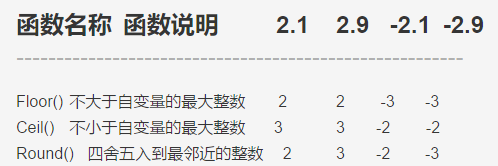但是我试了一下会显示这样的错误，error C2065: ‘round’ : undeclared identifier，（目前还不知道为什么会这样，还没去查）然后我就四舍五入关系写了一个，需要保留的小数，调用函数就可以啦。
/*自定义函数四舍五入*/
int round(float f)
{
if ((int)f+0.5>f)
return (int)f;
else
return (int)f + 1;
}


2题外话****我还用到了绝对值函数，下面是我结合课本所学知识和网上查到，都是我测试过的，比网上找的靠谱哦！（因为关于头文件跟调用函数）
首先，绝对值里可以是任意的两个变量哦，下面是我在处理大物时写的
E=(fabs(e_Zhong_Ever-e0))/1.60e-19;	cout<<"  E相对误差："<<E*100<<'%'<<endl;

在处理大物实验数据时，通常在求解相对误差时需要用到绝对值函数
首先，绝对值里可以是任意的两个变量哦，下面是我在处理大物时写的
E=(fabs(e_Zhong_Ever-e0))/1.60e-19;	cout<<"  E相对误差："<<E*100<<'%'<<endl;

下面都是我在结合所学知识以及网上查找测试之后写的，因为网上有的介绍的头文件与相应的函数是不匹配的。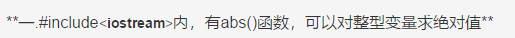# include<iostream>
//# include<cmath>
using namespace std;

int main()
{int a;
//double b;
a=abs(223-3);
//b=fabs(223.8-3.5);
cout<<"a="<<a<<endl;
//cout<<"b="<<b<<endl;
return 0;
}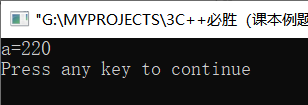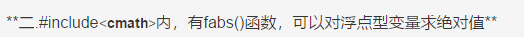# include<iostream>
# include<cmath>      //浮点型需要求绝对值
using namespace std;

int main()
{int a;
double b;
a=abs(223-3);
b=fabs(223.8-3.5);
cout<<"a="<<a<<endl;
cout<<"b="<<b<<endl;
return 0;
}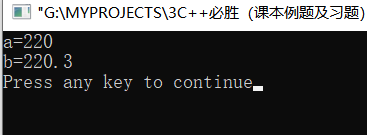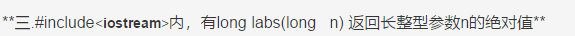这个就不举例啦，不常用到。


展开全文• 密立根油滴实验数据处理（平衡法和动态法） 平衡法 //平衡法 #include <iostream> #include <cmath> using namespace std; int main() { float q,t,r,u,x,x1,x2,n1,e1,n2,p1,E; float p2=981,g=9....
密立根油滴实验数据处理（平衡法和动态法）

平衡法

//平衡法
#include <iostream>
#include <cmath>
using namespace std;
int main()
{
float q,t,r,u,x,x1,x2,n1,e1,n2,p1,E;
float p2=981,g=9.794,l=1.6e-3,b=8.22e-3,p=0.10133e6,d=5.00e-3,n=1.83e-5,p0=1.293,e0=1.602e-19;
cout<<"t下降,U平衡"<<endl;
cin>>t>>u;
p1=p2-p0;
r=sqrt(9*n*l/(2*p1*g*t));
x1=((n*l)/(t*(1+b/(p*r))));
x2=x1*x1*x1;
x=sqrt(x2);
q=18*3.1415926535898*x*d/(sqrt(2*p1*g)*u);
n1=q/(1.602e-19);
if(n1-int(n1)>=0.5)
{n2=int(n1)+1;}
else if(n1-int(n1)<0.5||n1-int(n1)>=0)
{n2=int(n1);}
else
{cout<<"error";}
e1=q/n2;
E=(e1-e0)/e0;
cout<<"r="<<r<<endl;
cout<<"q="<<q<<endl;
cout<<"n="<<n1<<endl;
cout<<"e="<<e1<<endl;
cout<<"误差："<<E<<endl;
return 0;
}


动态法

#include <iostream>
#include <cmath>
using namespace std;
int main()
{
float q,t,r,u,x,x1,x2,n1,e1,n2,p1,tg,k,E;
float p2=981,g=9.794,l=1.6e-3,b=8.22e-3,p=0.10133e6,d=5.00e-3,n=1.83e-5,p0=1.293,e0=1.602e-19;
cout<<"t下降,t上升,U上升"<<endl;
cin>>tg>>t>>u;
p1=p2-p0;
r=sqrt(9*n*l/(2*p1*g*tg));
k=18*3.1415926*d*l/(sqrt(2*p1*g));
x1=((n)/((1+b/(p*r))));
x2=x1*x1*x1;
x=sqrt(x2);
q=k*x*(1/t+1/tg)*sqrt(l/tg)*(1/u);
n1=q/(1.602e-19);
if(n1-int(n1)>=0.5)
{n2=int(n1)+1;}
else if(n1-int(n1)<0.5||n1-int(n1)>=0)
{n2=int(n1);}
else
{cout<<"error";}
e1=q/n2;
E=(e1-e0)/e0;
cout<<"k="<<k<<endl;
cout<<"r="<<r<<endl;
cout<<"q="<<q<<endl;
cout<<"n="<<n1<<endl;
cout<<"e="<<e1<<endl;
cout<<"误差："<<E<<endl;
return 0;
}

具体固定参数可按需自行修改，如下落距离l。

展开全文• 密立根油滴实验报告 密立根油滴实验实验报告 密立根(R.A.Millikan)在 1910-1917年的七年间，致力于测量微小油滴上 所带电荷的工作，这即是著名的密立根油滴实验，它是近代物理学发展过程中 具有重要意义的实验。...
• 大学物理实验报告系列之密立根油滴实验大学物理实验报告【实验名称】 密立根油滴实验 【实验目的】1．了解密立根油滴实验仪的结构以及利用油滴测定电子电荷的设计思想和方法。2．通过对带电油滴在重力场和静电场中...
• 本学期《大学物理实验》重修课程须完成2部分内容：1.“智慧树”线上课程(占期末总成绩50%)2.虚拟仿真实验(占期末总成绩50%)1“智慧树”线上课程学生须通过“智慧树网”或“知到”APP完成全部内容。包括全部视频的...
• 文档介绍：密立根油滴实验的计算机仿真在物理学史上,确定电子的荷质比并进而测定电子电量,是一件极有意义的工作。美国著名的实验物理学家密立根从1907年开始,在总结前人实验的基础上,着手电子电荷量的测量研究,后来...